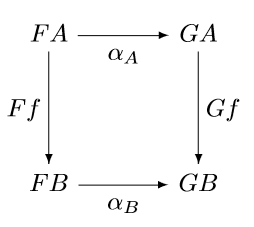# Natural transformations

The ladder of abstractions in category theory starts with categories, then functors, then natural transformations. Unfortunately, natural transformations don’t seem very natural when you first see the definition. This is ironic since the original motivation for developing category theory was to formalize the intuitive notion of a transformation being “natural.” Historically, functors were defined in order to define natural transformations, and categories were defined in order to define functors, just the opposite of the order in which they are introduced now.

A category is a collection of objects and arrows between objects. Usually these “arrows” are functions, but in general they don’t have to be.

A functor maps a category to another category. Since a category consists of objects and arrows, a functor maps objects to objects and arrows to arrows.

A natural transformation maps functors to functors. Sounds reasonable, but what does that mean?

You can think of a functor as a way to create a picture of one category inside another. Suppose you have some category and pick out two objects in that category, A and B, and suppose there is an arrow f between A and B. Then a functor F would take A and B and give you objects FA and FB in another category, and an arrow Ff between FA and FB. You could do the same with another functor G. So the objects A and B and the arrow between them in the first category have counterparts under the functors F and G in the new category as in the two diagrams below.A natural transformation α between F and G is something that connects these two diagrams into one diagram that commutes.The natural transformation α is a collection of arrows in the new category, one for every object in the original category. So we have an arrow αA for the object A and another arrow αB for the object B. These arrows are called the components of α at A and B respectively.

Note that the components of α depend on the objects A and B but not on the arrow f. If f represents any other arrow from A to B in the original category, the same arrows αA and αB fill in the diagram.

Natural transformations are meant to capture the idea that a transformation is “natural” in the sense of not depending on any arbitrary choices. If a transformation does depend on arbitrary choices, the arrows αA and αB would not be reusable but would have to change when f changes.

The next post will discuss the canonical examples of natural and unnatural transformations.

## 4 thoughts on “Natural transformations”

1. Pseudonym

Possibly my favourite intuition of what is a “natural transformation” is from Haskell.

Suppose you have two Haskell functors, F and G, with map functions that I’ll call mapF and mapG.

Consider the following function:

`eta :: forall a. F a -> G a`

Then the parametricity theorem (a.k.a. the “free theorem”; see Wadler’s paper “Theorems for Free”) states that for all functions f:

`mapG f . eta = eta . mapF f`

This is precisely the commutation square of a natural transformation! Intuitively, the function eta can’t look “inside” the functor, so it must commute with a type substitution. Or, to put it another way, that’s what the “forall” in the type of eta actually means.

2. Kit Joyce

Some criticisms of data analysis practices such as Andrew Gelman’s “garden of forking paths” and Simmons/Nelson/Simonsohn “researcher degrees of freedom” relate to the arbitrariness of choices made in the analysis (cutpoints/binning, exclusions etc). Are you aware of any attempts to formalise this notion of arbitrariness in data analysis in terms of natural transformations? I feel there could be some way to connect the two ideas but no idea what categories and functors would be involved.

3. Kit: That’s a very interesting question. In fact there is a paper that uses category theory to try to tell whether a statistical model makes sense.

“What is a statistical model?” by Peter McCullagh. The Annals of Statistics, 2002, Vol. 30, No. 5, 1225–1310

4. June Apple

Is it helpful to think this way?
A and B are content. f transforms content. Functors F, G are two different wrappers for the content and f. The commuting diagram expresses the condition that transforming the content be independent of changing the wrapper. It is possible to change the wrapper first F(A) -> G(A) and then the content G(A) -> G(B) or change the content first F(A) -> F(B) and then the wrapper F(B) -> G(B).